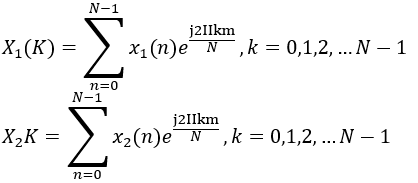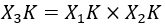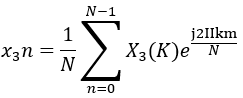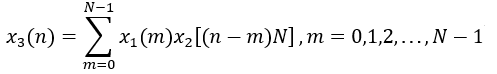Goseeko blog# What is Circular Convolution?

The Circular Convolution can be performed using two methods: concentric circle method and matrix multiplication method. Assuming x1(n) and x2(n) as two finite sequences of length N.

Now let us consider X1(K) and X2(K) as the inverse DFTs of sequences x1(n) and x2(n). The DFT for the sequences isLet  x3(n) be one more sequence with DFT X3(K). The relation between the three finite duration sequences is given asAfter taking inverse discrete Fourier transform of above sequences we haveThe above equation can also be written as## Methods of Circular Convolution

The two basic methods normally used for circular convolution are −

• Concentric circle method,
• Matrix multiplication method.

Concentric Circle Method

We firstly assume two finite length sequences x1(n) and x2(n). Now using the below steps we can easily circular convolve the two sequences.

• Firstly, we take two concentric circles. Then at the circumference of this circle we plot N samples of x1(n) with equal distance between each point. This plot is then done in an anticlockwise direction.
• In the inner circle we plot N samples of x2(n) in clockwise direction. Then the starting point is kept the same as in x1(n).
• Then we multiply the corresponding samples. The result of multiplication is added to obtain the final value.
• At last the value of the inner circle is to be rotated in an anticlockwise direction with one sample at a time.

Matrix Multiplication Method

Here we represent the given sequences x1(n) and x2(n) in matrix form.

• The NXN matrix is formed by repeating one of the sequences. This is then achieved by making a circular shift of one sample.
• Then the Second sequence forms a column matrix.
• Finally the result of circular convolution is calculated by multiplying these two matrices.

Interested in learning about similar topics? Here are a few hand-picked blogs for you!